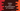# Python program to print all combinations of three numbers## Introduction :

In this python programming tutorial, we will learn how to print all combinations of three different numbers. The program will take three numbers as input from the user and print out the possible combination of the three numbers.

## Algorithm :

We will use three loops to print out the combination of all digits.

1. Take the numbers as input from the user. Store these values in three different variables.
2. Append all these numbers to a list.
3. Using three for loops, print out the combination of these numbers.
4. All these three loops indicate the three-position while printing out the numbers. So, we will print any value if the current index of these loops is not the same.

### Python program to find the combinations of three numbers :

The python program will look as below :

``````# 1
num1 = int(input("Enter the first number : "))
num2 = int(input("Enter the second number : "))
num3 = int(input("Enter the third number : "))

# 2
num_list = []
num_list.append(num1)
num_list.append(num2)
num_list.append(num3)

# 3
for i in range(0, 3):
for j in range(0, 3):
for k in range(0, 3):
if(i != j & j != k & k != i):
print("[{} {} {}]".format(
num_list[i], num_list[j], num_list[k]))``````

You can also download this program from here.

### Explanation :

The commented numbers in the above program denote the step numbers below:

1. Ask the user to enter the first, second and the third number. Read the integer numbers and store them in num1, num2 and num3 variables.
2. Create one empty list num_list. Append all these three numbers to the list.
3. Run three for loops. All these loops will run for three times: from index 0 to 2. Inside all of these loops, check if the current index is different or not for all. If yes, print out the number of the specific position from the list.

### Sample Output :

``````Enter the first number : 1
Enter the second number : 2
Enter the third number : 3
[1 2 3]
[1 3 2]
[2 1 3]
[2 3 1]
[3 1 2]
[3 2 1]

Enter the first number : 8
Enter the second number : 9
Enter the third number : 3
[8 9 3]
[8 3 9]
[9 8 3]
[9 3 8]
[3 8 9]
[3 9 8]``````

### Conclusion :

We have learned how to print all combinations of three numbers in python. We can also print all combinations of any numbers in a similar way. We can also solve this problem by using a while loop instead of a for loop. Try to run the program and drop one comment below if you have any queries.